# Basic Constructions

CONSTRUCT A COPY OF A SEGMENT
1. ﻿Select the Segment toolfrom the menu and create a segment to the right of segment AB﻿ (make sure its longer than AB) by clicking twice anywhere to the right of AB. Creating Segment CD.
2. ﻿Select the Compass tool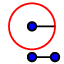: ﻿﻿ and select point B, then select point A. Click point C to center the circle at point C.
3. ﻿Select the Intersect tool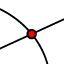from the menu and select the intersection created by the circle and segment CD, creating point E at the intersection.
4. Select the Distance or Length tool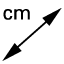from the menu. Select points A and B, then select points C and E. Be sure the two measures are congruent.
5. Use the text tool to type your first and last name as well as your student ID.
SEGMENT BISECTOR / PERPENDICULAR BISECTOR CONSTRUCTION
1. Select the Compass tool from the menu ﻿﻿and select point A, then select point B. Click point A to center the circle at A.
2. Select the Compass tool: ﻿﻿ and select point B, then select point A. Click point B to center the circle at B.
3. Select the Intersect tool from the menu and select the two intersections created by the circles, creating points C and D at the intersections.
4. Select the Segment tool from the menu and click on point C, then on point D. Creating an intersection of segment AB and segment CD.
5. Select the Intersect tool from the menu and click on the intersection of segment AB and CD. Creating point E. **Point E is the midpoint of segment AB and Segment CD. **
6. Select the Distance or Length tool from the menu. Select point A and Point E. Select point B and E. Be sure the two measures are congruent.
7. Use the text tool to type your first and last name as well as your student ID.

## Construct a Congruent Angle

1. Select the Compasstool from the menu ﻿﻿and select vertex B, then select point C. Click point B to center the circle at B.
2. Select the Intersecttool from the menu and select the circle created in step 1, and segment BA, creating point F at the intersection.
3. Select the Compasstool from the menu ﻿﻿and select vertex B, then select point C. Click point D to center the circle at D.
4. Select the Intersecttool from the menu and select the circle centered at D and the ray DE, creating point G at the intersection.
5. Select the Compasstool from the menu ﻿﻿and select point C, then select point F. Click point C to center the circle at C.
6. Select the Compasstool from the menu ﻿﻿and select point C, then select point F. Click point G to center the circle at G.
7. Select the Intersecttool from the menu and click on circle D and circle G. Creating point H at the intersection of the two circles.
8. Select the Ray tool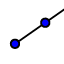from the menu and click points D and then point H. Creating ray DH.
9. Measure the angle using the angle tool from the menu by selecting the angle tool from the menu, clicking point G, then vertex D, then point H. Make sure the measures of angle B and angle D are
10. Use the text toolto type your first and last name as well as your student ID.

## Construct an Angle Bisector

1. Select the Circle with Center through Point ﻿tool from menu. Click on point B and anywhere between point B and C. Creating point D on segment BC.
2. Select the Intersecttool from the menu. Select the circle created from step 1, and segment AB creating point E at the intersection.
3. Select the Circle with Center through Point ﻿tool from the menu. Select point D then point E, creating a circle with a radius congruent to DE.
4. Select the Circle with Center through Point ﻿tool from the menu. Select point E then point D, creating a circle with a radius congruent to DE.
5. Select the Intersecttool from the menu. Click the circles created from step 2 and 3, and creating point F and point G at the intersections.
6. Select the Raytool from the menu. Click vertex B and either point G OR F. Creating Ray BG, or BF which bisects angle ABC.
7. Select the angletool from the menu. Click points D, then B, then F, creating angle DBF.
8. Select the angletool from the menu. Click points F, then B, then E, creating angle FBE.
9. Compare angles DBF and FBE to make sure they're congruent.

## Constructing Parallel Lines

1. Select the linetool from the menu. Click point A and anywhere below segment BC.
2. Select the intersecttool from the menu and select line AD and segment BC. Creating vertex E and angle CEA.
3. Select the Circle with Center Though Point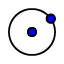tool from the menu. Click on vertex E and anywhere between points E and C on segment BC creating point F. *be sure that the circle created does not go beyond point A.
4. Select the intersecttool from the menu and select circle E then line AD. Creating point G between segment EA.
5. Select the Compasstool from the menu and click vertex E, then point F, then click point A to center the circle at point A.
6. Select the intersecttool from the menu and select circle A then line AD. Creating point I above point A.
7. Select the Compasstool from the menu and click point G, then point F, then click point I to center the circle at point I and create an intersection of circle A and circle I.
8. Select the intersecttool from the menu and select circle A, then circle I. Creating a points K and L at the intersections.
9. Select the Linetool from the menu and click points A and K, creating line AK that is parallel to segment BC.

## Construct Perpendicular Lines Through a Point ON the Line/Segment

1. Select the Circle with Center Through Pointtool from the menu and select point A on segment BC then click somewhere below segment BC, creating circle A. *** the circle's radius should NOT extend past point B***.
2. Select the Intersecttool from the menu and select circle A and segment BC creating points E and F on segment BC.
3. Select the Circle with Center Through Pointtool from the menu and select point E, then point F, creating circle E.
4. Select the Circle with Center Through Pointtool from the menu and select point F, then point E, creating circle F.
5. Select the Intersecttool from the menu and select circle E and circle F creating points G and H at the intersections of the two circles.
6. Select the Linetool from the menu and click on points G and H to create a line perpendicular to segment BC that passes through point A.
7. Select the Angletool from the menu and select point F, Vertex A, and point G. Verify that angle GAF is exactly 90º.

## Construct Perpendicular Lines Through a Point NOT on the Line/Segment

1. Select the Circle with Center Through Pointtool from the menu and select point A on segment BC then click somewhere below segment BC, creating circle A that intersects BC in two places.
2. Select the Intersecttool from the menu and select circle A and segment BC creating points E and F on segment BC.
3. Select the Circle with Center Through Pointtool from the menu and select point E, then point F, creating circle E.
4. Select the Circle with Center Through Pointtool from the menu and select point F, then point E, creating circle F.
5. Select the Intersecttool from the menu and select circle E and circle F creating points G and H at the intersections of the two circles.
6. Select the Line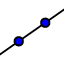tool from the menu and click on points G and H to create a line perpendicular to segment BC that passes through point A.
7. Select the Intersecttool from the menu and select Line GH and segment BC creating point I.
8. Select the Angletool from the menu and select point F, point I, and point G. Verify that angle FIG is exactly 90º.

## Construction of an Inscribed Equilateral Triangle

1. Select the Point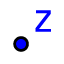tool from the menu and select anywhere on circle A.
2. Select the Circle with Center Through Pointtool from the menu and click point B, then point A. Creating a circle with radius AB, centered at point B. This creates two intersections, click one of the two intersections (with the tool still selected) then point A, creating a congruent circle to circle B. Repeat the process, creating points C, D, E, F and G as you go completely around circle A.
3. Select the Polygontool from the menu and select points, B, D, F, and B again.
4. Select the angle tool from the menu and select point F, then B, then D. Repeat with points B, D, and F, and points D, F, and B. Confirm that each angle measures 60º.
5. Select the Distance or Lengthtool from the menu and click points B, then D, and point D, then F, and point F then B. Confirm that each side length is congruent.

## Construction of an Inscribed Regular Hexagon

1. Select the Pointtool from the menu and select anywhere on circle A.
2. Select the Circle with Center Through Pointtool from the menu and click point B, then point A. Creating a circle with radius AB, centered at point B. This creates two intersections, click one of the two intersections (with the tool still selected) then point A, creating a congruent circle to circle B. Repeat the process, creating points C, D, E, F and G as you go completely around circle A.
3. Select the Polygontool from the menu and select points, B, C, D, E, F, G, and B again.
4. Select the Distance or Lengthtool from the menu and measure all the sides of the polygon. Confirm that each segment has the same length.

## Inscribe a Square in a Circle

1. Select the LINEtool from the menu and click point A and anywhere on circle A.
2. Select the Pointtool from the menu and select the intersection of circle A and Line AB, creating point C on circle A.
3. Select the Circle with Center Through Pointtool from the menu and click point C, then point B. Creating a circle with radius BC, centered at point B. With thetool still selected, click point C then point B. Creating a circle with radius CB, centered at point C.
4. Select the Intersecttool from the menu and click on Circle B, then on circle C. Creating points D and E.
5. Select the Segmenttool from the menu and click points D and E, creating segment DE.
6. Select the Intersecttool from the menu and click on Circle A, then on segment DE. Creating points F and G on circle A.
7. Select the Polygontool from the menu and select points, B, F, C, G, and then B again.
8. Select the angle tool from the menu and select point F, then C, then G. Repeat with points C, G, and B, and points G, B, and F. Confirm that each angle measures 90º.
9. Select the Distance or Lengthtool from the menu and measure all the sides of the polygon. Confirm that each segment is congruent.